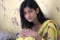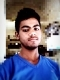## Sudoku Solving algorithmsUpdated on 16-Jun-2020 07:04:00
In this section, we will try to solve the famous number maze problem called Sudoku. Sudoku is a 9 x 9 number grid, and the whole grid are also divided into 3 x 3 boxes There are some rules to solve the Sudoku.We have to use digits 1 to 9 for solving this problem.One digit cannot be repeated in one row, one column or in one 3 x 3 box.Using the backtracking algorithm, we will try to solve the Sudoku problem. When some cell is filled with a digit, it checks whether it is valid or not. When it is ... Read More

## Subset Sum ProblemUpdated on 16-Jun-2020 07:09:28
In this problem, there is a given set with some integer elements. And another some value is also provided, we have to find a subset of the given set whose sum is the same as the given sum value.Here backtracking approach is used for trying to select a valid subset when an item is not valid, we will backtrack to get the previous subset and add another element to get the solution.Input and OutputInput: This algorithm takes a set of numbers, and a sum value. The Set: {10, 7, 5, 18, 12, 20, 15} The sum Value: 35 Output: All ... Read More

## Solving Cryptarithmetic PuzzlesUpdated on 16-Jun-2020 07:16:15
In the crypt-arithmetic problem, some letters are used to assign digits to it. Like ten different letters are holding digit values from 0 to 9 to perform arithmetic operations correctly. There are two words are given and another word is given an answer of addition for those two words.As an example, we can say that two words ‘BASE’ and ‘BALL’, and the result is ‘GAMES’. Now if we try to add BASE and BALL by their symbolic digits, we will get the answer GAMES.NOTE &minuns; There must be ten letters maximum, otherwise it cannot be solved.Input and OutputInput: This algorithm will ... Read More

## Rat in a Maze ProblemUpdated on 16-Jun-2020 07:23:16
In this problem, there is a given maze of size N x N. The source and the destination location is top-left cell and bottom right cell respectively. Some cells are valid to move and some cells are blocked. If one rat starts moving from start vertex to destination vertex, we have to find that is there any way to complete the path, if it is possible then mark the correct path for the rat.The maze is given using a binary matrix, where it is marked with 1, it is a valid path, otherwise 0 for a blocked cell.NOTE: The rat can ... Read More

## N Queen ProblemUpdated on 16-Jun-2020 07:51:36
This problem is to find an arrangement of N queens on a chess board, such that no queen can attack any other queens on the board.The chess queens can attack in any direction as horizontal, vertical, horizontal and diagonal way.A binary matrix is used to display the positions of N Queens, where no queens can attack other queens.Input and OutputInput: The size of a chess board. Generally, it is 8. as (8 x 8 is the size of a normal chess board.) Output: The matrix that represents in which row and column the N Queens can be placed. If the ... Read More

## M-Coloring ProblemUpdated on 16-Jun-2020 07:58:12
In this problem, an undirected graph is given. There is also provided m colors. The problem is to find if it is possible to assign nodes with m different colors, such that no two adjacent vertices of the graph are of the same colors. If the solution exists, then display which color is assigned on which vertex.Starting from vertex 0, we will try to assign colors one by one to different nodes. But before assigning, we have to check whether the color is safe or not. A color is not safe whether adjacent vertices are containing the same color.Input and ... Read More

## Hamiltonian CycleUpdated on 16-Jun-2020 08:05:28
In an undirected graph, the Hamiltonian path is a path, that visits each vertex exactly once, and the Hamiltonian cycle or circuit is a Hamiltonian path, that there is an edge from the last vertex to the first vertex.In this problem, we will try to determine whether a graph contains a Hamiltonian cycle or not. And when a Hamiltonian cycle is present, also print the cycle.Input and OutputInput: The adjacency matrix of a graph G(V, E). Output: The algorithm finds the Hamiltonian path of the given graph. For this case it is (0, 1, 2, 4, 3, 0). This ... Read More

## Z AlgorithmUpdated on 16-Jun-2020 08:13:31
This algorithm is named Z Algorithm because, in this algorithm, we need to create a Z array. The size of the Z array is the same as the text size. This array is used to store the length of longest possible substring starting from the current character of the main string. At first, the pattern and the main text are concatenated with a special symbol which is not present in the text and pattern. If the P is pattern and T is the main text, then after concatenation, it would be P$T (Assuming$ is not present in the P ... Read More

## Trie of all SuffixesUpdated on 15-Jun-2020 19:10:41
From the text, we can generate all suffixes to make a tree structure. We know that every pattern that presents in the text, must be a prefix of one of the possible suffix in the text. By building Trie of all suffixes, we can find any substring in linear time. Every suffix is ending with string terminating symbol. From each node if there is any path, it moves forward, otherwise returns that pattern is not found.For this algorithm, the time complexity is O(m+k), where the m is the length of string and k is the frequency of the pattern in ... Read More

## Suffix ArrayUpdated on 15-Jun-2020 19:15:53
From a given string, we can get all possible suffixes. After sorting the suffixes in lexicographical order, we can get the suffix array. Suffix arrays can also be formed using suffix trees. By using the DFS traversal of suffix trees, we can get suffix arrays. Suffix arrays are helpful to find suffixes in linear time. We can also find substrings using suffix array by using binary search type procedure.The time complexity is O(m log n)Input and OutputInput: Main String: “BANANA”, Pattern: “NAN” Output: Pattern found at position: 2AlgorithmfillSuffixArray (text, suffArray)Input: The main stringOutput: The array of suffixesBegin    n := text Length ... Read More